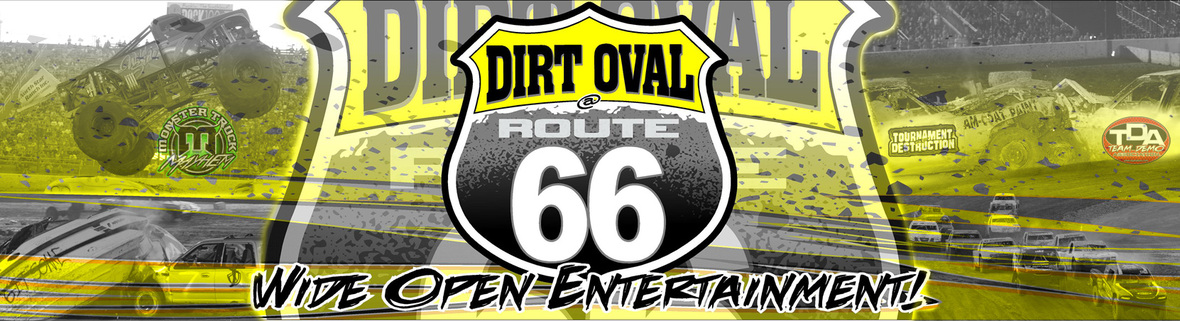table div table+table div table td,table.module-1{width:100%;padding:0}table div table+table div table{width:100%;float:none;margin-left:auto;margin-right:auto;padding:0}table div table+table div table a{border:0 none;text-decoration:none}table div table+table div table img{width:100%!important;border:0 none;text-decoration:none}/* styles */

Team Demolition Derby and Dirt Oval at Route 66 shine the spotlight on the Athletes of Special Olympics

Saturday night, the "Five-O at the Dirty O" Tournament of Destruction lights up the Dirt Oval at Route 66 Raceway! It is an official Law Enforcement Torch Run fundraising event benefiting Special Olympics Illinois.
Advanced ticket purchase strongly recommended due to increased regional and national attendance.

3 WAYS TO GET TICKETS:

Buy now @ TeamDemo.com
Call 815-740-8000
At the Dirt Oval office
Zero processing fees with in-person purchase.
Kids 2yrs & under are FREE

8 Team Demolition Derby races | Figure-8 Cars | Figure-8 Trucks | Vehicle Displays | Fireworks | Police Car Race/* styles */ It's a critical night for the points battles across Team Demo and Figure-8 racing. All the top spots are on the line! Parking opens at 4pm for Tailgating | Action begins at 7:15pm15 Departments | 15 Laps | Speeding is Legal

Reckless Driving could be a Ticket to victory in the first-ever Five-O at the Dirty O police car race all to benefit the awesome athletes of Special Olympics! Each race team was required to raise a minimum \$500 donation in order to compete. As of July 20, that goal has been shattered. The teams have combined for an incredible total of over \$35,000 in donations!
Contributions are still welcome before and during the event!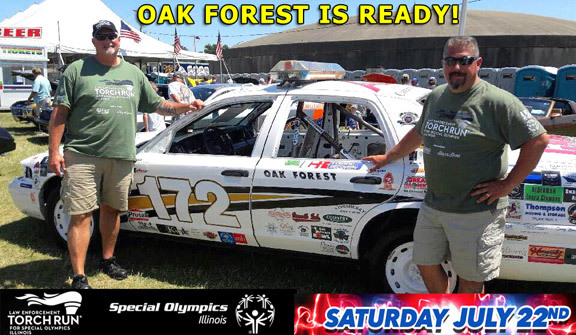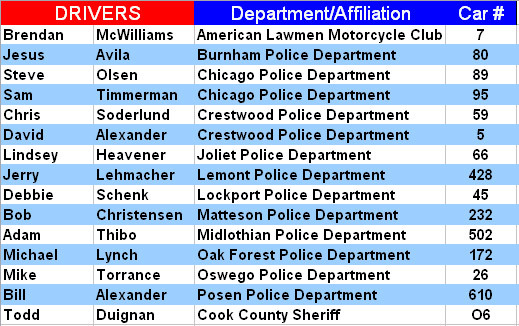table.module-9{width:91.7%;padding:0}table div table+table+table+table+table+table+table+table+table+table div table{width:91.7%;float:none;margin-left:auto;margin-right:auto;padding:0}table div table+table+table+table+table+table+table+table+table+table div table a{border:0 none;text-decoration:none}table div table+table+table+table+table+table+table+table+table+table div table img{width:100%!important;border:0 none;text-decoration:none}table div table+table+table+table+table+table+table+table+table+table div table td{width:100%;padding:0}/* styles */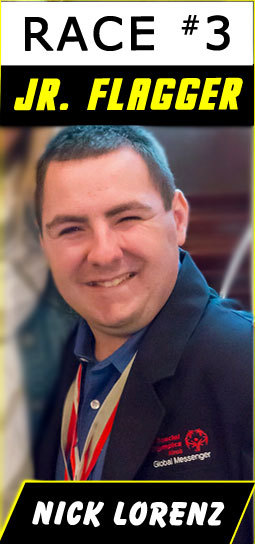Special Olympics Athletes will be active throughout race day!

Nick Lorenz is a Special Olympics Illinois Athlete, competing in bowling, bocce, swimming, track, softball and basketball over the course of more than 10 years. He’s an avid Chicago sports fan and soon-to-be Team Demo fan for life. He will throw the Green Flag to kick off the racing action!

Bree Bogucki is also an Athlete, Global Messenger and accomplished singer with performances logged across the world. Her goal is to continue educating others on Acceptance for All.

Images courtesy Special Olympics IL and nFocus Photos.

 table.module-12{width:85.47%;padding:0}table div table+table+table+table+table+table+table+table+table+table+table+table+table div table{width:85.47%;float:none;margin-left:auto;margin-right:auto;padding:0}table div table+table+table+table+table+table+table+table+table+table+table+table+table div table a{border:0 none;text-decoration:none}table div table+table+table+table+table+table+table+table+table+table+table+table+table div table img{width:100%!important;border:0 none;text-decoration:none}table div table+table+table+table+table+table+table+table+table+table+table+table+table div table td{width:100%;padding:0}/* styles */

4th Annual Monster Truck Mayhem

 table div table+table+table+table+table+table+table+table+table+table+table+table+table+table+table div table td,table.module-14{width:100%;padding:0}table div table+table+table+table+table+table+table+table+table+table+table+table+table+table+table div table{width:100%;float:none;margin-left:auto;margin-right:auto;padding:0}table div table+table+table+table+table+table+table+table+table+table+table+table+table+table+table div table a{border:0 none;text-decoration:none}table div table+table+table+table+table+table+table+table+table+table+table+table+table+table+table div table img{width:100%!important;border:0 none;text-decoration:none}/* styles */
 /* styles */ Monster Trucks return on Saturday, August 5th! The stacked lineup includes last year's winners, the "monstah lobstah" CRUSHSTATION and the Chevy powerhouse OVER BORED! The super-sized dump truck DIRT CREW; crazy four-wheeler QUAD CHAOS; iconic AVENGER, and more are ready to conquer the Dirt Oval. Plus, the pure mayhem of trailer racing! Adults: \$20 | Kids: \$12 Get your tickets at DirtOval66.com
 table div table+table+table+table+table+table+table+table+table+table+table+table+table+table+table+table+table div table{width:100%;padding:0}table div table+table+table+table+table+table+table+table+table+table+table+table+table+table+table+table+table div table table{padding:0;float:left!important;width:51.886%!important}table div table+table+table+table+table+table+table+table+table+table+table+table+table+table+table+table+table div table table+table td,table div table+table+table+table+table+table+table+table+table+table+table+table+table+table+table+table+table div table td{padding-left:0;padding-right:0}table div table+table+table+table+table+table+table+table+table+table+table+table+table+table+table+table+table div table table td{padding-left:0;padding-right:20px}table div table+table+table+table+table+table+table+table+table+table+table+table+table+table+table+table+table div table table+table{float:left!important;width:48.114%!important}/* styles */

Team Demolition Derby #ThrowbackThursday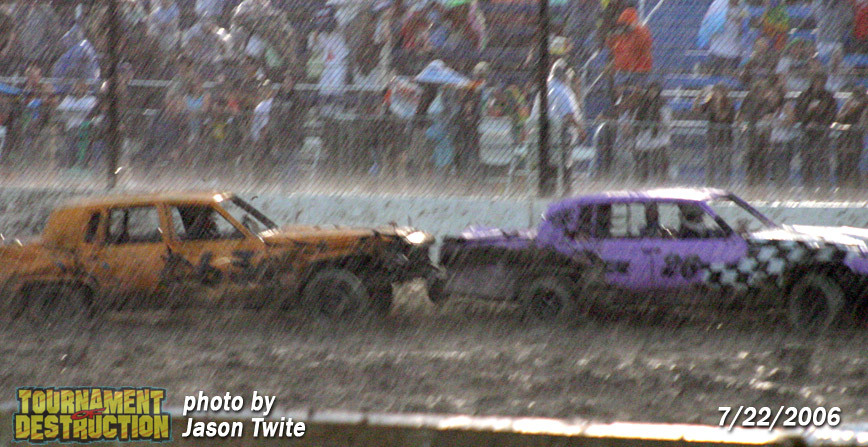/* styles */ July 22, 2006: 11 years ago, the Van Allen brothers had barely made a dent on their Team Demo careers under the purple guise of Team Xtreme. On this night, torrential rain hit hard in Round 1. Team Xtreme stayed afloat, pulled off the shocking upset over Orange Crush, then sunk Junkyard Dogs in the Semi-Finals. When the skies cleared for the Finals, Team Xtreme met a roadblock in the form of Road Rage. The intimidating Road Rage had a 4-on-3 advantage and showed no mercy in their route to clinch the 2006 Championship. A memorable night for many reasons, one that was ultimately Team Xtreme's only Finals appearance. The Van Allen's switched to Stranglehold in 2008, but never found the winning combination. The Finals drought extended until May 2011 when Jay Van Allen, as part of Full Throttle, defeated brother Zac and Mean Green Machine en route to a runner-up finish against Reckoning.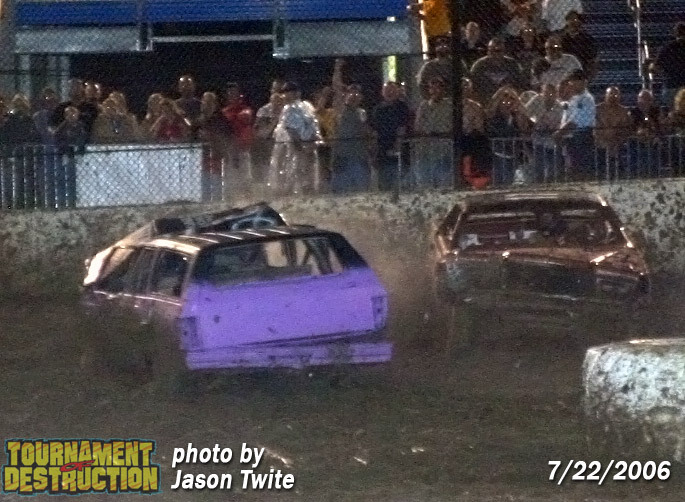Fair share of Wreckage coming next week in Lake County

 table.module-22{width:98.11%;padding:0}table div table+table+table+table+table+table+table+table+table+table+table+table+table+table+table+table+table+table+table+table+table+table+table div table{width:98.11%;float:none;margin-left:auto;margin-right:auto;padding:0}table div table+table+table+table+table+table+table+table+table+table+table+table+table+table+table+table+table+table+table+table+table+table+table div table a{border:0 none;text-decoration:none}table div table+table+table+table+table+table+table+table+table+table+table+table+table+table+table+table+table+table+table+table+table+table+table div table img{width:100%!important;border:0 none;text-decoration:none}table div table+table+table+table+table+table+table+table+table+table+table+table+table+table+table+table+table+table+table+table+table+table+table div table td{width:100%;padding:0}/* styles */
 /* styles */ The Dirt Oval's brand of entertainment and excitement heads North to the Lake County Fair in Grayslake on July 26th for the Eve of Destruction! The on-track action isn't limited to just the older crowd. Kids get to suit up and pulverize some plastic in the Power Wheels demolition derby!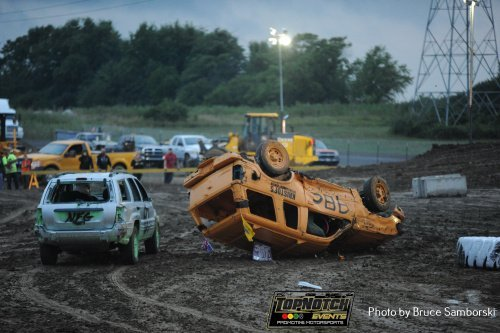Lawn Mower demo derby

Trucks, Cars and School Buses racing (i.e. smashing) on the Figure-8

Rules and Driver registration details are at WePromoteRacing.com
Tickets are FREE with paid admission to the fair.

 table.module-25{width:76.04%;padding:0}table div table+table+table+table+table+table+table+table+table+table+table+table+table+table+table+table+table+table+table+table+table+table+table+table+table+table div table{width:76.04%;float:none;margin-left:auto;margin-right:auto;padding:0}table div table+table+table+table+table+table+table+table+table+table+table+table+table+table+table+table+table+table+table+table+table+table+table+table+table+table div table a{border:0 none;text-decoration:none}table div table+table+table+table+table+table+table+table+table+table+table+table+table+table+table+table+table+table+table+table+table+table+table+table+table+table div table img{width:100%!important;border:0 none;text-decoration:none}table div table+table+table+table+table+table+table+table+table+table+table+table+table+table+table+table+table+table+table+table+table+table+table+table+table+table div table td{width:100%;padding:0}/* styles */
 table.module-26{width:98.49%;padding:0}table div table+table+table+table+table+table+table+table+table+table+table+table+table+table+table+table+table+table+table+table+table+table+table+table+table+table+table div table{width:98.49%;float:none;margin-left:auto;margin-right:auto;padding:0}table div table+table+table+table+table+table+table+table+table+table+table+table+table+table+table+table+table+table+table+table+table+table+table+table+table+table+table div table a{border:0 none;text-decoration:none}table div table+table+table+table+table+table+table+table+table+table+table+table+table+table+table+table+table+table+table+table+table+table+table+table+table+table+table div table img{width:100%!important;border:0 none;text-decoration:none}table div table+table+table+table+table+table+table+table+table+table+table+table+table+table+table+table+table+table+table+table+table+table+table+table+table+table+table div table td{width:100%;padding:0}/* styles */
 table div table+table+table+table+table+table+table+table+table+table+table+table+table+table+table+table+table+table+table+table+table+table+table+table+table+table+table+table div table{width:100%;padding:0}table div table+table+table+table+table+table+table+table+table+table+table+table+table+table+table+table+table+table+table+table+table+table+table+table+table+table+table+table div table table{padding:0;float:left!important;width:50%!important}table div table+table+table+table+table+table+table+table+table+table+table+table+table+table+table+table+table+table+table+table+table+table+table+table+table+table+table+table div table td{padding-left:5px;padding-right:5px}table div table+table+table+table+table+table+table+table+table+table+table+table+table+table+table+table+table+table+table+table+table+table+table+table+table+table+table+table div table table+table{float:left!important;width:50%!important}table div table+table+table+table+table+table+table+table+table+table+table+table+table+table+table+table+table+table+table+table+table+table+table+table+table+table+table+table div table table td{padding-left:0;padding-right:20px}table div table+table+table+table+table+table+table+table+table+table+table+table+table+table+table+table+table+table+table+table+table+table+table+table+table+table+table+table div table table+table td{padding-left:0;padding-right:0}/* styles */
 Like   Tweet   Pin   +1   in